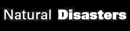# The Birth of Number Theory - Research Article from Natural Disasters and Man-Made Disasters

This encyclopedia article consists of approximately 6 pages of information about The Birth of Number Theory.
 This section contains 1,618 words (approx. 6 pages at 300 words per page) View a FREE sample## Overview

Number theory is the mathematical study of the properties of numbers and the relationships between numbers. The study of prime numbers, for example, is a focus of much of number theory, and Fermat's famous Last Theorem was, until recently, one of the major unsolved problems in the theory of numbers. A Greek mathematician, Diophantus of Alexandria (third century A.D.), did some of the very first work in number theory, and, in so doing, set the stage for nearly two millennia of future work.

## Background

A prime number is a number that can be evenly divided by only itself and the number one. There is no way to know with certainty when prime numbers were first discovered, but it was certainly near the dawn of mathematics. They have been known of for at least 2,500 years, and undoubtedly much longer...

 This section contains 1,618 words (approx. 6 pages at 300 words per page) View a FREE sample